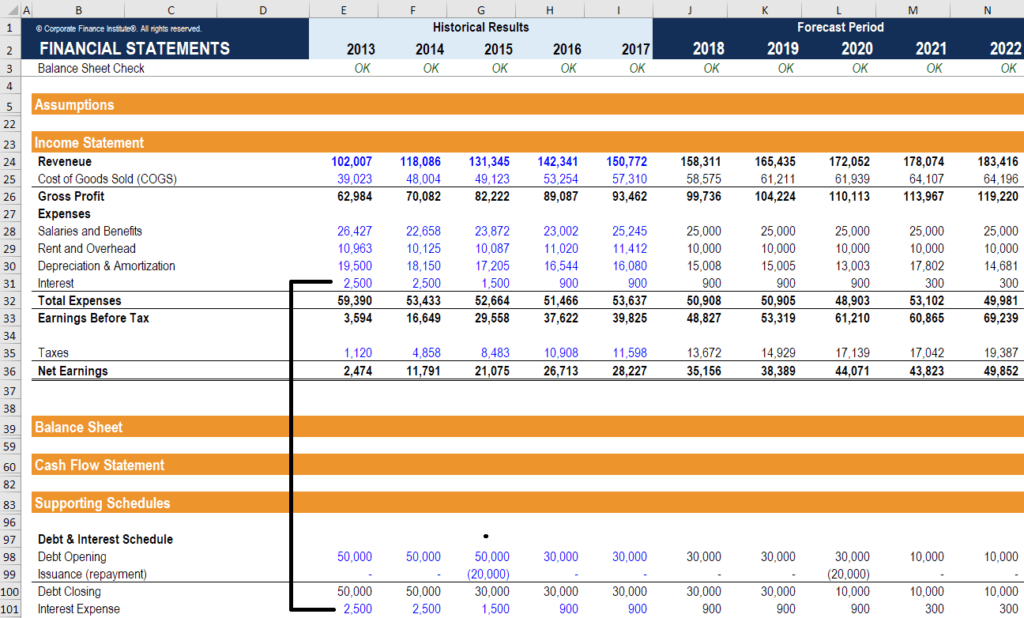# Interest Expense

Interest incurred when company finances through debt

## Interest Expense

Interest expense is one of the core expenses found in the income statement. A company must finance its assets either through debt or equity. With the former, the company will necessarily incur an expense related to the cost of borrowing. Understanding a company’s interest expense helps to understand its capital structure and financial performance.

Interest is often found as a separate line item below EBIT (Earnings Before Interest and Taxes). Alternatively, some companies may list interest in the SG&A section, depending on their accounting practices.

Most commonly, interest expense arises out of company borrowing money. However, another transaction that generates interest expense is the use of capital leases. When a firm leases an asset from another company, the lease balance generates an interest expense that appears on the income statement.

### Where does the expense appear on the income statement?

Below is an example of where interest expense appears on the income statement:Interest is found in the income statement, but can also be calculated through the debt schedule. The schedule should outline all the major pieces of debt a company has on its balance sheet, and the balances on each period opening (as shown above). This balance is multiplied by the interest rate to find the expense. Capital leases are not typically found in the debt schedule.

Learn how to calculate interest expense and debt schedules in CFI’s financial modeling courses.

### Interest Expense formula

Here is the formula to calculate interest on the income statement:

Interest Expense = Average Balance of Debt Obligation x Interest Rate

### EBIT and EBT

Interest is deducted from Earnings Before Interest and Taxes (EBIT) to arrive at Earnings Before Tax (EBT).

EBIT is also known as Operating Profit, while EBT is also known as Pre-Tax Income or Pre-Tax Profit.

Interest, therefore, is typically the last item before taxes are deducted to arrive at net income.

### Income tax deductibility (tax shield)

Interest is a tax-deductible item on the income statement. Thus, there is a tax savings, referred to as the tax shield.

For example:

If a company has zero debt and EBT of \$1 million (with a tax rate of 30%), their taxes payable will be \$300,000.

If the same company takes on debt and has an interest cost of \$500,000 their new EBT will be \$500,000 (with a tax rate of 30%), and their taxes payable will now be only \$150,000.

### More resources

We hope this has been a helpful guide. To keep learning and developing your accounting skills we highly recommend these additional CFI resources, in addition to our library of free finance and accounting courses offered online.

• The 3 financial statements
• Income statement template
• How to link the 3 statements
• Balance sheet overview